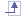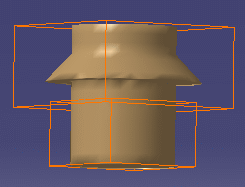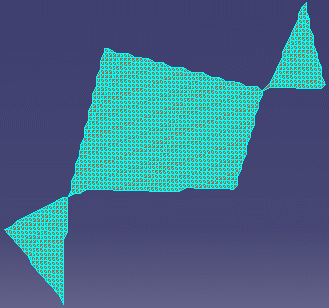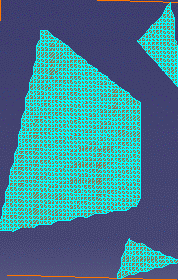# Glossary

## Aactivate This function is used to define a particular portion of a cloud for further operations.

## Ccell A mesh may consist of several cells (i.e. sub-clouds): for example, the mesh below consists of two cells.characteristic line They are particular lines corresponding for instance to curvature variations (fillets start/end) or sharp edges, ...

## Fflipping edge An edge common to two triangles of a mesh may be flipped, that is rotated,
to respect the shape a sharp edge of the tessellated part.

## Mmesh A mesh consists of a set of meshal faces (triangles) which represent the surface of a 3D model.
A triangulation is computed to describe the neighborhood relation of all points.

A mesh can be used to check the quality of the points, or can be processed in other applications.

A mesh may present some irregularities such as:

• Corrupted triangles, i.e. triangles that have the same vertex twice,
• Duplicated triangles, i.e. triangles that share the same three vertices,
• Non-manifold edges, i.e. edges shared by more than two triangles,
• Non-manifold vertices, i.e. vertices shared by two or more connected shells.

A mesh may also present some structural problems such as:

• Orientation problems, i.e. all the triangles are not oriented in the same direction,
• Isolated triangles, i.e. triangles belonging to small connected areas of the mesh,• Disconnected zones, i.e. the mesh is made of several disconnected zones,• Triangles with long edges.

## Rremove This function deletes physically points from the cloud of points. The points cannot be recovered.

## S